# Elementary Statistics for Stochastic Modeling

To understand the principles and practice of stochastic modeling, you need to have an effective working knowledge of a few simple concepts from statistics. If you have taken a statistics class, you should find the following to be a quick review. If not, then I hope you will find my approach here to be relatively qualitative and therefore quickly grasped.

# The Probability Density Function

The Probability Density Function (PDF) expresses the probability of a continuous random variable (CRV) taking on a value between any two points in the range of that variable. The probability of an event occuring between any two values, a and b, of the CRV is given as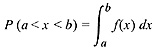where f(x) is the probability distribution function.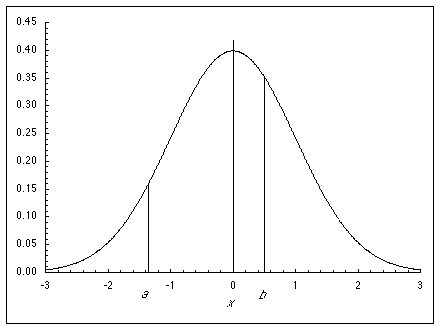Probability is valid for a CRV on an interval only. In other words, the probability of any single value of x is zero. For example, if the possible values of the net present value (NPV) of an investment in a new pump are distributed as a CRV, the probability of the NPV being exactly $1 million is zero. The probability of the NPV being between$0.9 million and \$1.1 million, however, is some finite number greater than zero and can be determined from the PDF for the NPV of the pump investment. In a qualitative sense, the PDF does show the comparative distribution of the likelihood of x taking on a specific value. In the curve above, for example, you can clearly see by inspection that an outcome of zero is more likely than an outcome of +1 or –2.

# The Cumulative Distribution Function

The Cumulative Distribution Function (CDF) expresses the cumulative probability of a CRV taking on a value between the lower bound and a point in the range of that variable. The probability of an event occuring between any two values, a and b, of the CDF is given as

P(a < x < b) = g(b) – g(a)

where g(x) is the cumulative distribution function.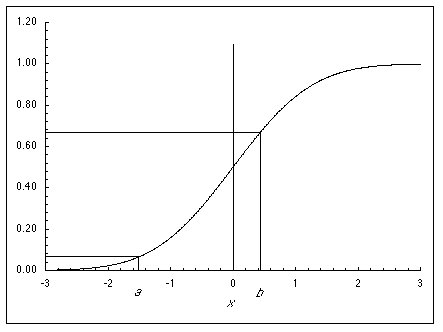For example, in the graph above, the probability of x taking on a value between a and b is approximately 0.67 – 0.07 = 0.60. The following relationships hold between the PDF, f(x), and the CDF, g(x):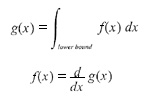# The Normal Distribution

The Normal distribution (ND) is the most common and useful of the continuous distribution functions. It occurs often in nature because of the central limit theorem. The ND is a two-parameter distribution characterized by its mean, µ, and its standard deviation, . The Standardized Normal distribution is an ND with µ= 0 and  = 1. The continuous random variable is scaled as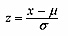The Standardized Normal PDF is shown here:The Standardized Normal CDF is shown here:In stochastic modeling, the normal distribution is best used to model variables whose bounds are distant from the range of interest.

# The Log-Normal Distribution

The log-normal distribution (ND) is the continuous random variable resulting from transforming the normal distribution with the exponential function, i.e. if f(x) is the normal probability density function, then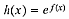where h(x) is the log-normal PDF. The log-normal distribution is a two-parameter distribution described by its mean, µ, and its standard deviation, . The log-normal PDF and CDF are shown below, respectively:In stochastic modeling, the log-normal distribution is best used to model asymmetric variables with a bound near the range of interest.

# The Triangular Distribution

The triangular distribution is a simple distribution that has no real source in nature. It is most, if not exclusively, useful for stochastic modeling rather than statistical analysis because of its artificial nature. It is a three-parameter distribution described by two zero-probability endpoints and a most probable point (mode). The intensity of the mode can be proven to be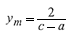The triangular PDF and CDF are shown below, respectively: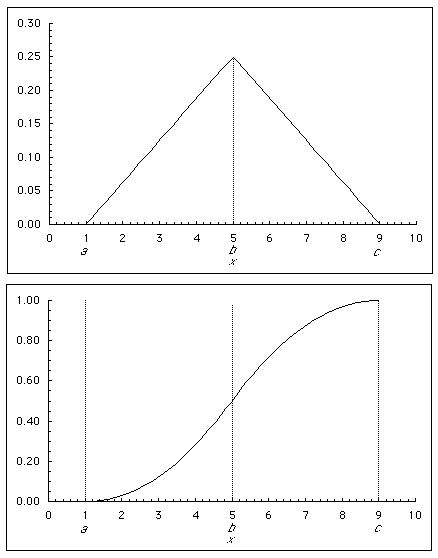In stochastic modeling, the triangular distribution is best used to model variables with both upper and lower bounds near the range of interest. The distribution does not need to be, and frequently is not, symmetric.

# The Central Limit Theorem

The central limit theorem is one of the most important concepts in statistics and is a strong driver of the results one sees in stochastic modeling. It states

If x is distributed with mean, µ, and standard deviation, , then the mean obtained from a random sample of size n will have a distribution that approaches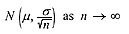A corollary of the central limit theorem can be stated as

If x is distributed with mean, µ, and standard deviation, , then the SUM(xi) obtained from a random sample of size n will have a distribution that approachesNote that the central limit theorem contains no assumption about the distribution that is sampled. It can be normal, log-normal, or something else.

The Central Limit Theorem is illustrated below: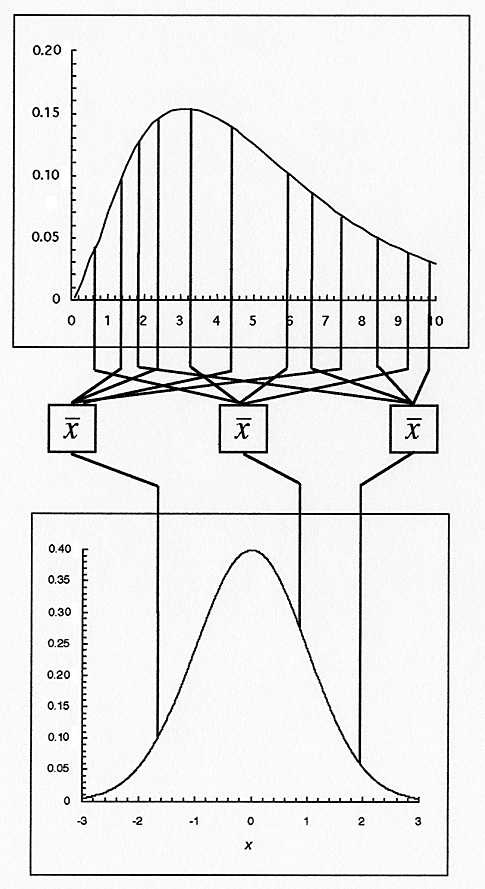# A Useful Result of the Central Limit Theorem

In stochastic modeling, the central limit theorem will drive output variables toward normal distributions. Said another way, the sum of a large number of random variables will be normally distributed regardless of the distributions of the individual random variables.

This corollary is illustrated below: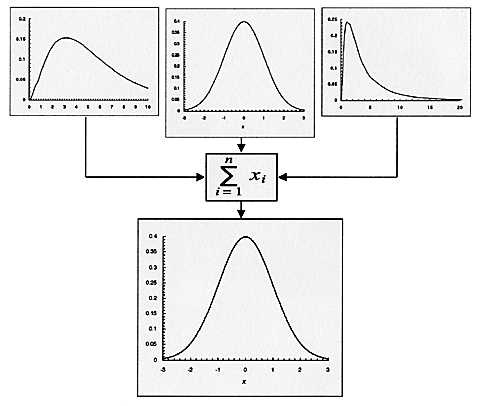Measurements of macro phenomena tend to be normally distributed because they are the sum of many micro distrubutions.

This site uses Akismet to reduce spam. Learn how your comment data is processed.